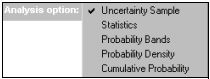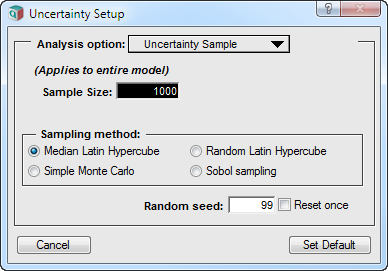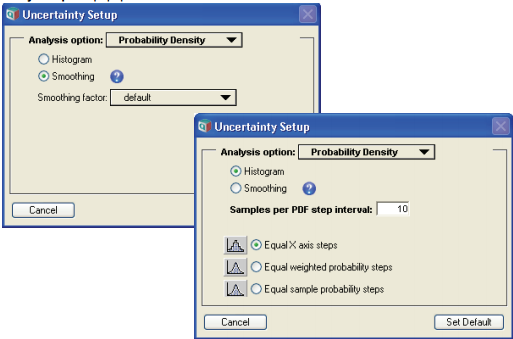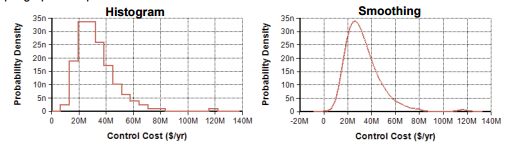# Uncertainty Setup dialog

(Redirected from Uncertainty Setup Dialog)

Release: 4.6  •  5.0  •  5.1  •  5.2  •  5.3  •  5.4  •  6.0  •  6.1  •  6.2  •  6.3  •  6.4

Use the Uncertainty Setup dialog to inspect and change options to compute and display uncertain quantities. To open the Uncertainty Setup dialog, select Uncertainty Options from the Result menu or Control+u. To set values for a specific variable, select the variable before opening the dialog. It contains five menu options:Uncertainty sample lets you set the sample size and sampling method and applies to all variables in the model. The other four, Statistics. The others, Probability bands, Cumulative probability and Probability density, let you set options for each of those views for each variable or as the default for the entire model. It saves all settings with your model. See below for details on each item.

## Uncertainty sampleSample size: This number specifies how many runs or iterations Analytica performs to estimate probability distributions. Larger sample sizes take more time and memory to compute, and produce smoother distributions and more precise statistics. See Selecting the Sample Size for guidelines on selecting a sample size. In the Analytica Free Edition the sample size must be between 2 and 32,000. You can access this number in expressions in your models as the system variable SampleSize.

### Sampling method

The sampling method determines how to generate a random sample of the specified sample size, m, for each uncertain quantity. Analytica offers four options: Median Latin hypercube (the default), Random Latin hypercube, Monte Carlo, and Sobol (new in -Analytica 5.0). See Monte Carlo and probabilistic simulation for details.

### Random seed

The series of random numbers starts from this seed value when:

• A model is opened.
• The value in this field is changed.
• You check the Reset once box, and close the Uncertainty Setup dialog by clicking Accept or Set Default.

The value must be a number between 0 and 100,000,000 (108).

### Reset once

Check the Reset once box to produce the exact same series of random numbers.

## Statistics option

To select which statistics of the distribution to show when you select Statistics as the uncertainty view of a result.## Probability Bands option

To select which probability bands to display when you select Probability Bands as the uncertainty view of a result.## Probability density option

To change how probability density is estimated and drawn, when you select Probability Density as the uncertainty view of a result.Analytica estimates the probability density function, like other uncertainty views, from the underlying array of sample values for each uncertain quantity. The probability density is highly susceptible to random sampling variation and noise. Both histogramming and kernel density smoothing techniques are available for estimating the probability density from the sample. To reduce noise and variability it may be necessary to increase sample size (for details on selecting the sample size, see Selecting the Sample Size). This example compares the two methods on the same uncertain result:Histogram: The histogram estimation methods partition the space of possible continuous values into bins, and then tally how many samples land in each bin. The probability density is then equal to the fraction of the Monte Carlo sample landing in a given bin divided by the bin’s width. The average number of points landing in each bin determines both the smoothness of the resulting function and the resolution of the resulting plot. With more bins, a finer resolution is obtained, but since fewer points land in each bin, the amount of random fluctuation increases resulting in a noisier plot. The Samples per PDF step interval setting sizes the bin width to match the average number of points per bin. With larger sample sizes, you can increase the Samples per PDF step interval to achieve smoother plots, since more samples will land in each bin. A number approximately equal to the square root of sample size tends to work fairly well.

You can also control how the partitioning of the space of values is performed. When Equal X axis steps is used, the range of values from the smallest to largest sample point is partitioned into equal sized bins. With this method, all bins have the same width, but the number of points falling in each bin varies. When Equal weighted probability steps is used, the bins are sized so that each bin contains approximately the same fraction of the total probability. With this method, the fraction of the sample in each bin is nearly constant, but the width of each bin varies. When Equal sample probability steps is used, the bins are partitioned so that the number of sample points in each bin is constant, with the width of each bin again varying. Equal weighted probability steps and Equal sample probability steps are exactly equivalent when standard equally-weighted Monte Carlo or Latin Hypercube sampling is being used. They differ when the Sample Weighting system variable assigns different weights to each sample along the Run index, as is sometimes employed with importance sampling, logic sampling for posterior analysis, and rare-event modeling. See Importance weighting.

Probability density plots using the histogram method default to the Step chart type, which emphasizes the histogram and reveals the bin placements. When desired, this can be changed to the standard line style from the Graph Setup, see Chart Type tab.

Smoothing: The smoothing method estimates probability density using a technique known as Kernel Density Estimation (KDE) or Kernel Density Smoothing. This technique replaces each Monte Carlo sample with a Gaussian curve, called a Kernel, and then sums the curve to obtain the final continuous curve. Unlike a histogram, the degree of smoothness and the resolution of the plot are independent. The Smoothing factor controls the smoothness or amount of detail in the estimated PDF. The more info button next to the Smoothing radio control jumps to a page on the Analytica Wiki that elaborates in more detail on how kernel density smoothing works.

Due to the randomness of Monte Carlo sampling, estimations of probability density are often quite noisy. The Smoothing method can often provide smoother and more intuitively appealing plots than Histogram methods, but the averaging effects inherent in smoothing can also introduce some minor artifacts. In particular, Smoothing tends to increase the apparent variance in your result slightly, with a greater increase when the Smoothing factor is greater. This increase in variance is also seen as a decrease in the height of peaks. Sharp cutoffs (such as is the case with a Uniform distribution, for example) become rounded with a decaying tail past the cutoff point. And when positive-only distributions begin with a very sharp rise, the density estimate may get smoothed into a plot with a tail extending into the negative value territory.

## Cumulative probability option

To change how the cumulative probability values are drawn or to change their resolution, when you select Cumulative Probability as the uncertainty view of a result..Analytica estimates the cumulative distribution function, like other uncertainty views, from the underlying array of sample values for each uncertain quantity. As with any simulation-based method, each estimated distribution has some noise and variability from one evaluation to the next. Cumulative probability estimates are less susceptible to noise than, for example, probability density estimates.

The Samples per CDF plot point setting controls the average number of sample values used to estimate each point on the cumulative distribution function (CDF) curve, which ultimately controls the number of points plotted on your result.

The Equal X axis steps, Equal weighted probability steps and Equal sample probability steps control which points are used in plot of the cumulative probability. Equal X axis steps spaces points equally along the X axis. Equal weighted probability steps uses the sample to estimate a set of m+! fractiles (quantiles), Xp, at equal probability intervals, where p=0, q, 2q, ... 1, and q = 1/m. The cumulative probability is plotted at each of the points Xp, increasing in equal steps along the vertical axis. Points are plotted closer together along the horizontal axis in the regions where the density is the greatest. Equal sample probability steps plots one point each at each nth sample point, where n is the sample per CDF plot point, ignoring the weight on each sample point when they are weighted differently. The cumulative probability up to the nth point is estimated and plotted. Equal weighted probability steps and Equal sample probability steps are exactly equivalent unless unequal sample weighting is employed (see Importance weights).

## History

The Uncertainty Sample pane was reworked in Analytica 5.0. Prior to 5.0, an option for changing the pseudo-random generator method was shown. This was removed (if you really want to use a different random generator algorithm, you can still change this by changing the RandomType system variable).

The Sobol sampling option was added in Analytica 5.0.

The Kernel Density Smoothing option for probability density estimation was introduced in Analytica 4.4.